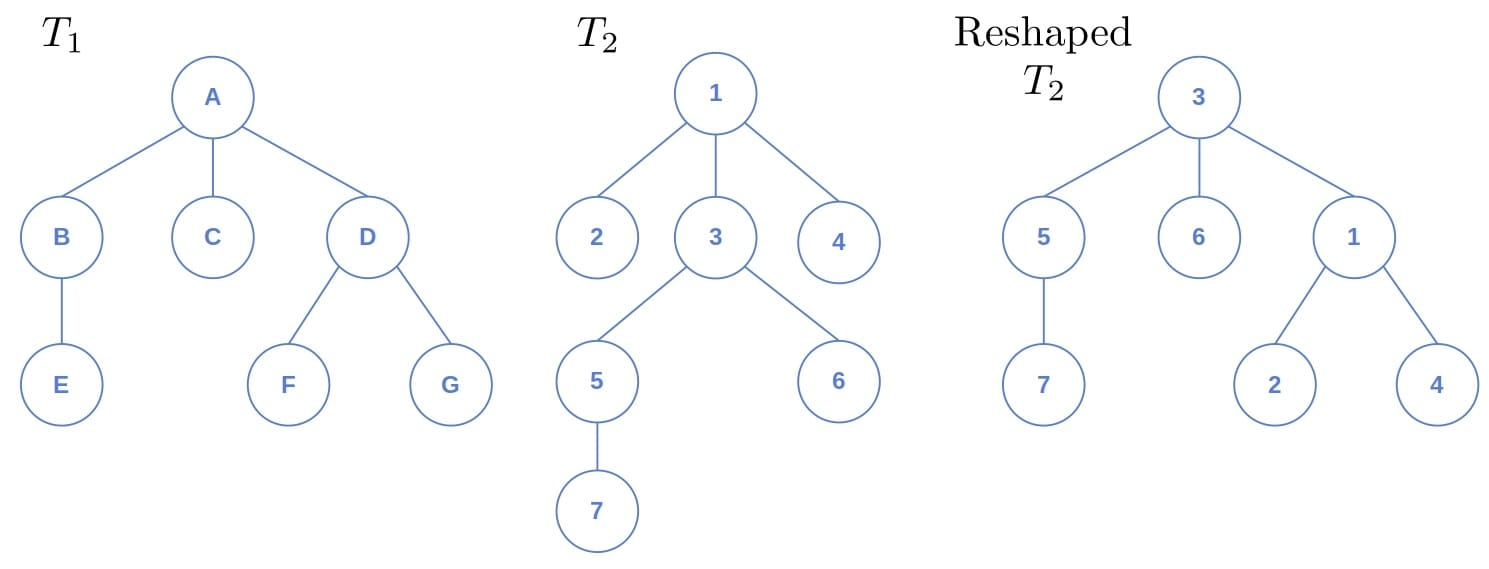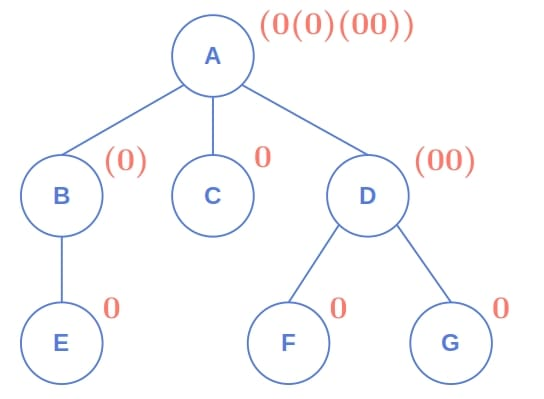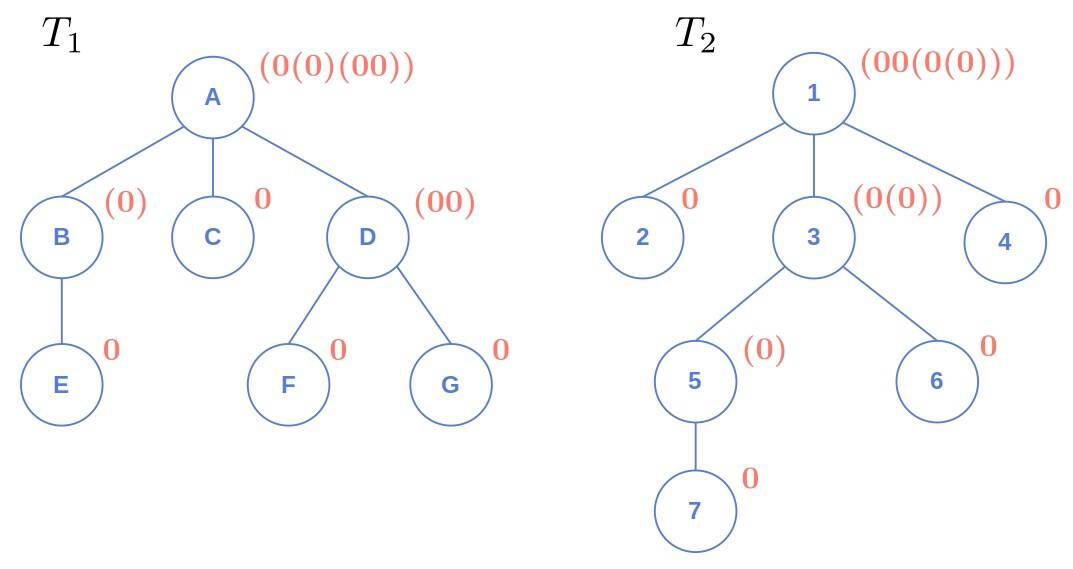## 1. Introduction

In this tutorial, we’ll talk about tree isomorphism and how to check if two trees are isomorphic.

## 2. Tree Isomorphism

Since trees are connected acyclic graphs, tree isomorphism is a special case of graph isomorphism.

The word isomorphism means the same shape. So, intuitively, we say that two trees are isomorphic if they have the same structure.

How do we prove isomorphism? We can redraw one tree to take the same shape as the other and match their nodes. For instance:That, however, isn’t always easy to do by hand, so we need an efficient algorithm and a precise definition of isomorphism.

### 2.1. Mathematical Definition

We can define isomorphism rigorously. Letandbe two trees, whereanddenote their nodes, andandtheir edges.

We say thatandare isomorphic if there exists a bijectionsuch that any two nodesare adjacent inif and only if they’re adjacent in:We call such a mappingthe isomorphism ofand.

Although we didn’t call it that way, we formulated the following isomorphism by reshapingin the previous example:## 3. Encoding

The idea is to encode each tree so that only the nodes of the trees sharing the same structure have the same encoding.

### 3.1. AHU Encoding

We’ll use a variation of the AHU (Aho, Hopcroft, Ullman) encoding scheme. It maps nodes to strings representing the structures of their subtrees.

If the nodeis a leaf, its AHU encodingis just the symbol 0.

In contrast, letbe a non-leaf node andits children. Its encoding is the string containing its children’s encodings in the non-descending order:where.

Finally,.

For example:### 3.2. Pseudocode

Here’s the pseudocode:Before we start encoding the nodes, we first need to traverse the tree to assign each node its level so that we can iteratively compute the AHU strings starting from the bottom. Using the postorder traversal, we can get the levels in time linear in the number of nodes.

## 4. Checking for Isomorphism

Now, we can extend the AHU encoding algorithm to isomorphism testing.

### 4.1. Root

Ifandare isomorphic, they’ll have the same AHU encoding only if their roots are at the same place in their shared structure:On the right, we have the same tree as on the left but rooted at a different node. As we see, their AHU encodings differ.

To fix this, we’ll first find the centers ofandand root the trees at them. If they have the same structure, their encodings, when rooted at the centers, will be the same.

Iforhave two centers, we’ll try all pairings.

### 4.2. Pseudocode

Here’s the pseudocode:So, we first find the centers. Then, we use the centers as the roots and try to match the encodings. If any combination gives a match, we’ll prove the isomorphism ofand. However, if we find no match, the trees aren’t isomorphic, so we return.

### 4.3. Improvement

However, we don’t use the fact that the nodes at the same levels will have the same AHU tuples in the case of isomorphism, so a mismatch at any level means we can start with the next pair of centers. In the previous algorithm, we computed the entire AHU encodings even though we can stop if we find the levels that differ.

Also, since we’ll compare the trees level by level, we can shorten the encodings as follows. After computing the strings for all the nodes at the level, we find the distinct encodings. Then, sort them, and we map the shortest unique string to 0, the second one to 1, and so on. When computing the encodings of the nodes at the level, we use the unique integers we assigned to the strings instead of the actual strings:freestar.config.enabled_slots.push({ placementName: "baeldung_incontent_2", slotId: "baeldung_incontent_2" });



After rooting the input tree at the centers, we check their heights because only trees with the same height can be isomorphic.

### 4.4. Complexity

In the worst case, both trees will have two centers. So, the while loop is executed at most four times. Its worst-case complexity is. Why? First, every node is processed exactly once in the entire algorithm. That amounts totime.

Second, ifis the trees’ arity, in each iteration, we sort at moststrings, each having no more thancharacters. Sinceis fixed and doesn’t grow with respect to, the overhead isper iteration. As there areiterations in the worst case, whereis the height, the total overhead is. Since, we get theoverhead.

Finally, the postorder traversal is also. So, the algorithm has thetime complexity in the worst case.

## 5. Conclusion

In this article, we showed how to check if two trees are isomorphic. We do this by rooting them at their centers and trying to match their AHU encodings. If the threes are indeed isomorphic, we’ll get a match.